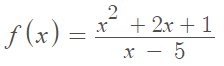# Wiggle Graph / Sign Graph: How to Draw One

A wiggle graph (or sign graph) is a segmented number line that describes where a function is increasing or decreasing. Derivatives give information about where a function is headed as well, so the wiggle graph is also a way to visualize the signs of a function’s derivative. It’s called a wiggle graph because it tells you where the function is “wiggling” towards.

## How to Draw a Wiggle Graph

Example question: Draw a wiggle graph for the function:Step 1: Find the first derivative. For this particular function, use the quotient rule.

1.Applying the quotient rule.
2.Simplifying

Step 2: Find the critical values for the function. Critical values are where the first derivative is equal to zero, or is undefined.

The derivative we calculated in Step 1,will be undefined if the denominator is equal to zero (because of division by zero). This happens when x = 5:We can factor the numerator to find out when the derivative will equal zero:When x = 11 or x = -1, the numerator will be zero, making the fraction equal zero.

Therefore, we have three critical values: x = -1, x = 5, and x = 11

Step 3: Draw a number line and label it with your critical values. Do not write any other numbers on the line. This doesn’t have to be drawn to scale:Step 4: Choose a number for each interval on the graph you drew in Step 3. We have four intervals in this example, labeled A, B, C, and D in this image:It doesn’t matter what numbers you choose, as long as you pick one from each interval. To make calculations relatively easy, I’ll pick -3, 1, 10 and 12.

Step 5: Plug the values you chose in Step 4 into the derivative from Step 1:Step 6: Label the wiggle graph with the signs of the first derivative. Add the label “f′(x)” to make it clear this wiggle graph is for the first derivative:The completed wiggle graph tells us that the function is increasing on the first interval, decreasing on the second and third intervals, and increasing on the fourth.

CITE THIS AS:
Stephanie Glen. "Wiggle Graph / Sign Graph: How to Draw One" From StatisticsHowTo.com: Elementary Statistics for the rest of us! https://www.statisticshowto.com/wiggle-graph-sign/